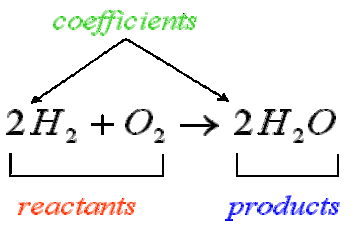## Amount of Reactants and Products

#### Learning Objective

• Construct a balanced chemical equation

#### Key Points

• To fully understand a chemical reaction, a balanced chemical equation must be written.
• Chemical reactions are balanced by adding coefficients so that the number of atoms of each element is the same on both sides.
• Stoichiometry describes the relationship between the amounts of reactants and products in a reaction.

#### Term

• stoichiometryThe field of chemistry that is concerned with the relative quantities of reactants and products in chemical reactions and how to calculate those quantities.

Chemical equations are symbolic representations of chemical reactions. The reacting materials (reactants) are given on the left, and the products are displayed on the right, usually separated by an arrow showing the direction of the reaction. The numerical coefficients next to each chemical entity denote the proportion of that chemical entity before and after the reaction. The law of conservation of mass dictates that the quantity of each element must remain unchanged in a chemical reaction. Therefore, in a balanced equation each side of the chemical equation must have the same quantity of each element.Chemical equationsA chemical equation shows what reactants are needed to make specific products. Reactions are balanced by adding coefficients so that there are the same number of atoms of each element on both sides of the reaction. So the left side of the equation, $2\text{H}_2 + \text{O}_2$, has four hydrogen atoms and two oxygen atoms, as does the right side of the equation, $2\text{H}_2\text{O}$.

## Stoichiometry

Stoichiometry is the field of chemistry that is concerned with the relative quantities of reactants and products in chemical reactions. For any balanced chemical reaction, whole numbers (coefficients) are used to show the quantities (generally in moles) of both the reactants and products. For example, when oxygen and hydrogen react to produce water, one mole of oxygen reacts with two moles of hydrogen to produce two moles of water.

In addition, stoichiometry can be used to find quantities such as the amount of products that can be produced with a given amount of reactants and percent yield. Upcoming concepts will explain how to calculate the amount of products that can be produced given certain information.

The relationship between the products and reactants in a balanced chemical equation is very important in understanding the nature of the reaction. This relationship tells us what materials and how much of them are needed for a reaction to proceed. Reaction stoichiometry describes the quantitative relationship among substances as they participate in various chemical reactions.